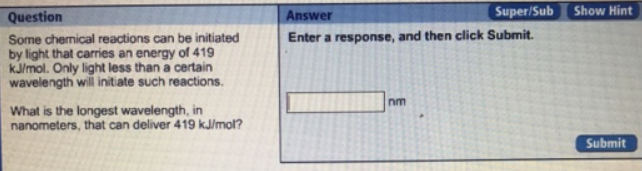# Problem: Some chemical reactions can be initiated by light that carries an energy of 419 kJ/mol. Only light less than a certain wavelength will initiate such reactions. What is the longest wavelength in nanometers that can deliver 419 kJ/mol? Convert the energy m kJ/mol to energy in J/photon. Use Planck's Equation to determine the frequency in hertz. Convert frequency to wavelength in nanometers h = 6.626 x 10^34 J

###### FREE Expert Solution
100% (4 ratings)###### Problem Details

Some chemical reactions can be initiated by light that carries an energy of 419 kJ/mol. Only light less than a certain wavelength will initiate such reactions. What is the longest wavelength in nanometers that can deliver 419 kJ/mol?

Convert the energy m kJ/mol to energy in J/photon. Use Planck's Equation to determine the frequency in hertz. Convert frequency to wavelength in nanometers h = 6.626 x 10^34 J